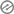# What is Arithmetic Operator in C# and How to Use Them?

## Arithmetic Operators

What is Arithmetic Operator in C# are called Binary operators because they work with two operands, we need to place the operator between the operands. For example, when we want to add two numbers, we use the plus (+) operator between the numbers that we want to add.

The arithmetic operators let you perform computation on numeric and strings. Below is the list of arithmetic operators that we have in C# language by Microsoft.

1. + (Plus)
2. – (Minus)
3. / (Devide)
4. * (Multiplication)
5. % (Modulus)

The above operations are famous enough to understand because you have seen them since your childhood. Some of them perform some extra operations that we will try to understand with program examples. Let us start with the first one.

### Plus Operator (+)

Unless you have been living under a rock, you would have an idea what this operator does but you also need to know this operator is special and perform one extra operation. This is used to perform addition between two or more numbers and also can concatenate two strings in C# programming language.

#### Lets see an example of addition

``````using System;
namespace Arithmetic_Operators
{
class Program
{
static void Main(string[] args)
{
var a = 12;
var b = 13;
var c = a + b;
Console.WriteLine("The value of c is {0}", c);
}
}
}
``````
##### Explaination

In the above example, two int variables have values in it and they are being added by the plus (+) operator. The output 25 you see on the screen is the addition of 12 and 13.

#### Let us see an example of concatenation

``````using System;
namespace Arithmetic_Operators
{
class Program
{
static void Main(string[] args)
{
var line1 = "I love C#";
var line2 = "Programming language";
Console.WriteLine(line1 + " " + line2);
}
}
}
``````
##### Explaination

In the above example, you can see there are two string variables line1 and line2. The line1 has a string inside it as “I love C#” and line2 has a string “Programming language”. In the output, you can see they are shown together as one string because the plus operator (+) is concatenating them as one single string. There is one single blank space that is also being concatenated in between those two strings.

### Minus Arithmetic Operator (-)

Just like its name, the operator is used to perform a subtraction between two numbers. How to use them, you can see in the example below.

#### Minus Operator Program

``````using System;
namespace Arithmetic_Operators
{
class Program
{
static void Main(string[] args)
{
var a = 12;
var b = 13;
var c = a - b;
Console.WriteLine("The value of c is {0}", c);
}
}
}
``````
##### Explaination

The program you can see above is the same as the plus operator, the only difference is the operator. This time we have used Minus operator (-) and substracted b from a. The output is printed on the console screen as -1.

### Divide Arithmetic Operator (/)

Before we start talking about divide operators first we need to understand the components of divide operation. I have created a chart for you guys, I hope you would like it. Look at the chart below.

As you can understand from the chart above. The number that divides the other number is called Divisor. The number that gets divided is called Dividend. The number of times a dividend divided completely is named Quotient. Once the Dividend is divided completely, if anything remains from the dividend is called Remainder.

The Divide Arithmetic Operator gives you Quotient

#### Divide Operator Program

``````using System;
namespace Arithmetic_Operators
{
class Program
{
static void Main(string[] args)
{
var a = 13;
var b = 5;
var c = a / b;
Console.WriteLine("The value of c is {0}", c);
}
}
}
``````

#### Explaination

The above program does the same divide operation as in the diagram. You can see the value comes out is 2 that is a Quotient.

### Multiplication Arithmetic Operator (*)

This operator is used to multiply two or more value together. Look at the program below.

#### Multiplication Operator Program

``````using System;
namespace Arithmetic_Operators
{
class Program
{
static void Main(string[] args)
{
var a = 13;
var b = 5;
var c = a * b;
Console.WriteLine("The value of c is {0}", c);
}
}
}
``````

### Modulus Arithmetic Operator (%)

We have seen the example of the divide operator and the diagram. The modulus operator provides you the remainder in that divide operation. If you do not understand what is the remainder, go look at the Diagram above.

#### Modulus Operator Program

``````using System;
namespace Arithmetic_Operators
{
class Program
{
static void Main(string[] args)
{
var a = 13;
var b = 5;
var c = a % b;
Console.WriteLine("The value of c is {0}", c);
}
}
}
``````
##### Explaination

When you divide 13 by 5 then it is divided completely two times and the remainder comes out as 3. The modulus operator is providing you Remainder in the program.

I hope you have understood all the operators. I know you might get confused between Modulus and Divide operator, I suggest you pay attention to the diagram and you will see the contrast.

See you next time guys, Stay Safe Stay Healthy!